The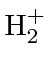Ion

The simplest molecule we can work with is theion. It has two nuclei (A and B) sharing one electron (1).is the distance between the two nuclei.

The lowest energy wavefunction can be thought of as a (anti)symmetric linear combination of an electron in the ground state near nucleus A and the ground state near nucleus B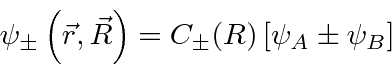where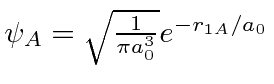is g.s. around nucleus A.andare not orthogonal; there is overlap. We must compute the normalization constant to estimate the energy.where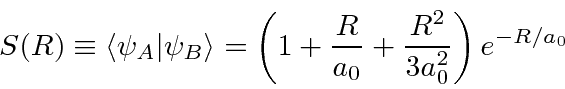These calculations are straightforward but tedious'' (Gasiorowicz).

We can now compute the energy of these states.We can compute the integrals needed.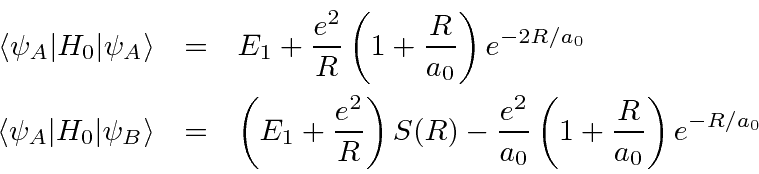We have reused the calculation ofin the above. Now, we plug these in and rewrite things in terms of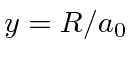, the distance between the atoms in units of the Bohr radius.The symmetric (bonding) state has a large probability for the electron to be found between nuclei. The antisymmetric (antibonding) state has a small probability there, and hence, a much larger energy.

The graph below shows the energies from our calculation for the space symmetricand antisymmetricstates as well as the result of a more complete calculation (Exact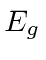) as a function of the distance between the protons. Our calculation for the symmetric state shows a minimum arount 1.3 Angstroms between the nuclei and a Binding Energy of 1.76 eV. We could get a better estimate by introduction some parameters in our trial wave function and using the variational method.

The antisymmetric state shows no minimum and never goes below -13.6 eV so there is no binding in this state.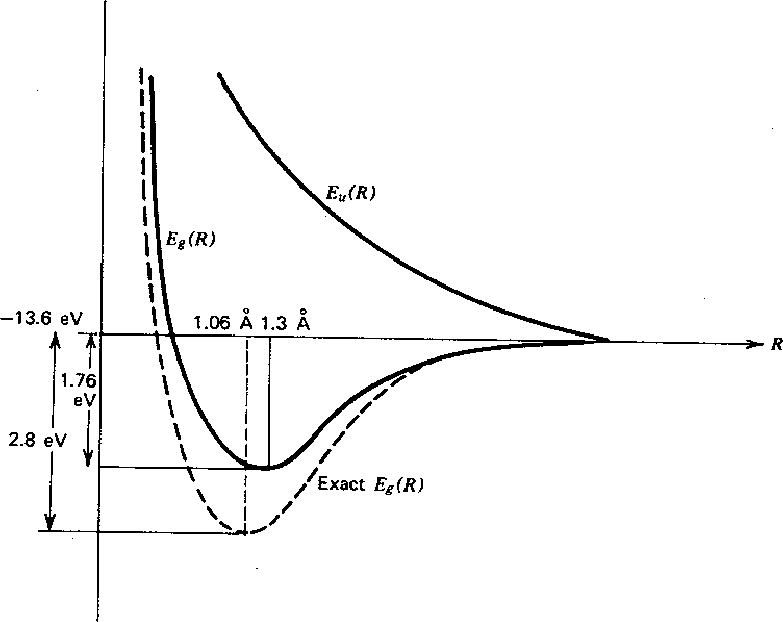By setting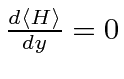, we can get the distance between atoms and the energy.

 Distance Energy Calculated 1.3 Å -1.76 eV Actual 1.06 Å -2.8 eV

Its clear we would need to introduce some wfn. parameters to get good precision.

Jim Branson 2013-04-22